Courses

# Short & Long Answer Question - Thermodynamics Class 11 Notes | EduRev

## JEE : Short & Long Answer Question - Thermodynamics Class 11 Notes | EduRev

The document Short & Long Answer Question - Thermodynamics Class 11 Notes | EduRev is a part of the JEE Course Physics For JEE.
All you need of JEE at this link: JEE

Q.1 What is a cyclic process? What is the change in internal energy of a system after it completes one cycle of such a process?

Answer: Any process in which the system returns to its initial state after undergoing a series of changes is known as a cyclic process. The change in internal energy after a complete cycle is zero because the system returns to its initial state.
Q.2 State the first law of thermodynamics.

Answer: According to the first law of thermodynamics, the amount of heat ΔQ absorbed by a system capable of doing mechanical work is equal to the sum of the increase in internal energy ΔU of the system and the external work ΔW done by the system. Mathematically, ΔQ=ΔU+ΔW=ΔU+PΔV.
Q.3 How does the internal energy of an ideal gas differ from that of real gas?

Answer: The internal energy of an ideal gas consists of only the kinetic energy of the particles. But for real gases it consists of both the kinetic as well as potential energies.
Q.4 Is the internal energy of a gas a function of pressure? Comment.

Answer: The internal energy of an ideal gas depends only on the temperature of the gas, while that of a real gas depends on the temperature and volume, which in turn is dependent on pressure.
Q.5 Out of a solid, liquid and gas of the same mass and at the same temperature, which one has the greatest internal energy? Which one least? Justify.

Answer: The gas has greatest internal energy because the potential energy (which is negative) of the molecules is very small. On the other hand, the (negative) potential energy of the molecules of a solid is very large; hence the internal energy of solid is least.
Q.6 When is the heat supplied to a system equal to the increase in its internal energy?

Answer: According to the first law of thermodynamics, ΔQ=ΔU+PΔV. If the heat is supplied in such a manner that the volume does not change (for isochoric change, ΔV=0). Then whole of the heat energy supplied to the system will increase internal energy only.
Q.7 A gas does work during isothermal expansion. What is the source of mechanical energy so produced?

Answer: By first law of thermodynamics, ΔQ=ΔU+ΔW. But for an isothermal process, ΔU=0, so ΔW=ΔQ. Thus the energy required for doing mechanical work during an isothermal process is obtained as heat by the gas from the surroundings.
Q.8 A gas does work during adiabatic expansion. What is the source of mechanical energy so produced?

Answer: By first law of thermodynamics, ΔQ=ΔU+ΔW. But for an adiabatic process, ΔQ=0,soΔW=−ΔU. Thus the source of energy required for doing mechanical work during adiabatic expansion is the internal energy of the gas itself.
Q.9 The temperature of a gas rises during an adiabatic compression, although no heat is given to the gas from outside. Why?

Answer: By   first   law   of   thermodynamics, ΔQ=ΔU+ΔW. For an adiabatic compression, ΔQ=0, so ΔU=−ΔW.That is work is done on the gas which increases its internal energy. Hence temperature of the gas rises.
Q.10 An ideal gas is compressed at constant temperature. Will its internal energy increase or decrease?

Answer: It will remain same because the internal energy of a gas depends only on its temperature.
Q.11 Cooling is produced when a gas at high pressure suddenly expands. Why?

Answer: During its expansion, the gas does work against high pressure. This decreases the internal energy and hence the temperature of the gas.
Q.12 When a gas is suddenly compressed, its temperature rises. Why?

Answer: Sudden compression of a gas is an adiabatic process. The work done in compressing the gas increases ' the internal energy of the gas. Hence the temperature of the gas rises.

Q.13 If an inflated tyre bursts, the air escaping out is cooled, why?

Answer: When the tyre bursts, there is an adiabatic expansion of air because the pressure of the air inside is sufficiently greater than the atmospheric pressure. During the expansion, the air does some work against the surroundings, therefore, its internal energy decreases, and as such temperature falls.
Q.14 Is it possible that there is change in temperature of a body without giving heat to it or taking heat from it?

Answer: Yes, for example, during an adiabatic compression temperature increases and in an adiabatic expansion temperature decreases, although no heat is given to or taken from the system in these changes.
Q.15 Is it possible that there is no increase in the temperature of a body despite being heated?

Answer: Yes, for example, during a change of state (from solid to liquid or from liquid to gas), the system takes heat, but there is no rise in temperature. Internal energy of the system increases in each case.
Q.16 Why does air pressure in a car tyre increase during driving?

Answer: During driving, as a result of the friction between the tyre and the road, the temperature of the tyre and hence that of air inside it, increases. Since the volume of air in the tyre remains constant, pressure of the air increases due to increase of temperature (Charle's law).
Q.17 Whose molecules: liquid water at 0C or ice at 0C have greater potential energy? Give reason.

Answer: Water molecules possess more potential energy. The heat given to melt the ice at 0C is used up in increasing the potential energy of water molecules formed at 0C.
Q.18 Why mechanical energy can be completely converted into heat energy but the whole of the heat energy cannot be converted into mechanical energy?

Answer: The whole of the mechanical energy can be absorbed by the molecules of the system in the form of their kinetic energy. This kinetic energy gets converted into heat. But the whole of the heat energy cannot be converted into work as a part of it is always retained by the system as its internal energy.
Q.19 During adiabatic changes, the volume of a gas is found to depend inversely on the square of its absolute temperature. Find how its pressure will depend on the absolute temperature.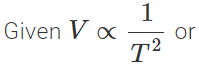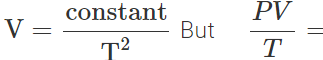constant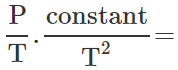constant or  P∝T3
Q.20 Does the mass of a body change when it is heated or cooled?

Answer: When a body is heated or cooled, it absorbs or releases energy and so its mass increases or decreases in accordance with the Einstein's relation:-E=mc2. As the speed of light c is very large, so the change in mass is extremely small.
Q.21 When air of the atmosphere rises up, it cools. Why?

Answer: When the air rises up, it expands due to the decrease in atmospheric pressure. It does work at the expense of its internal energy. So its temperature falls.
Q.22 Is the equation PV=RT valid for isothermal and adiabatic processes?

Answer: Yes, the equation PV=RT is valid for all types of the thermodynamical processes.
Q.23 If PV=RT is valid for all types of thermodynamical processes, what do the relations PV=a constant and PVγ= a  constant, signify?

Answer: The relations PV= a constant and PVγ=a  constant, are the relations between pressure and volume in the isothermal and adiabatic processes respectively. They are derived from the equation PV = RT.
Q.24 First law of thermodynamics does not forbid flow of heat from lower temperature to higher temperature. Comment.

Answer: First law of thermodynamics simply tells about the conversion of mechanical energy into heat energy and vice-versa. It does not put any condition as to why heat cannot flow from lower temperature to higher temperature.
Q.25 Can two isothermal curves intersect?
No. If two isotherms intersect, then this would mean that the pressure and volume of a gas are the same at two different temperatures. This is not possible.
Q.26 What is the significance of the area of closed curve on a P−V diagram?

Answer: The area gives the work done in a cyclic process.
Q.27 Can the work done during a cyclic process be zero?

Answer: Yes. The work done in a cyclic process is zero, if the process reverses exactly under similar conditions such that it retraces the same P−V diagram. In such a case, the area of the curve on a P−V diagram is zero and hence the work done is zero.
Q.28 How many specific heats does a gas possess?

Answer: A gas can possess infinite number of specific heats, depending upon the conditions of temperature and pressure. However, generally we consider only two specific heats, one at constant pressure and the other at constant volume.
Q.29 Why a gas has two principal specific heat capacities?
Or
Gases have two specific heats, but the solids and liquids possess only one specific heat. Why?

Answer: When the gases are heated, there occurs an appreciable change in their volume. So energy is required for expansion and we have two specific heats one at constant pressure and the other at constant volume. In case of solids and liquids, expansion is negligible, so they have only one specific heat i.e., at constant volume.
Q.30 Compare the formula CP−CV=R for an ideal gas with the thermodynamic relation ΔU=ΔQ−PΔV.

Answer: The equation ΔU=ΔQ−PΔV can be written as ΔQ=ΔU+PΔV This equation implies that when the heat ΔQ is given to an ideal gas, a part of it is used in increasing the internal energy (ΔU) and the remaining in doing work of expansion (PΔV). Similarly, the formula CP−CV=R can be written as CPΔT=CVΔT+RΔT This equation implies that when the heat CPΔT is given to an ideal gas at constant pressure, its one part is used in increasing the temperature or internal energy Undefined control sequence \Tau and the other part in doing work against external pressure (RΔT).
Q.31 A gas has two principal specific heats. Which one is greater and why?

Answer: Out of the many specific heats of a gas, two are of special significance : one when the gas is heated at constant volume and another when the gas is heated at constant pressure. These are known as the two principal specific heats of the gas and may be defined as follows :

(i) Molar specific heat at constant volume. It is defined as the amount of heat required to raise the temperature of 1 mole of a gas through 1C at constant volume. It is denoted by CV

(ii) Molar specific heat at constant pressure. It is defined as the amount of heat required to raise the temperature of 1 mole of a gas through 1C at constant pressure. It is denoted by CP. When a gas is heated at constant volume, all the heat supplied is used to increase the temperature or internal energy of the gas. When a gas is heated at constant pressure, the gas expands. It does work against the external pressure. The heat supplied is used in two ways :

(i) partly to increase the internal energy and

(ii) partly to do work against external pressure. So at constant pressure, an additional amount of heat equivalent to work done is utilized. Hence more amount of heat is required to increase the temperature of 1 mole of a gas at constant pressure than that at constant volume i.e., CP>CV. The difference between the two specific heats is equal to the amount of heat equivalent to the work performed by the gas during expansion at constant pressure.
Q.32 Of what physical significance is the difference between the two principal specific heat capacities and their ratio?

Answer: (i) The difference between the two principal specific heats is equal to the amount of heat equivalent to the work performed by the gas during expansion at constant pressure.

(ii) Knowing the specific heat ratio of a gas, we can determine the number of degrees of freedom and the atomicity of any gas.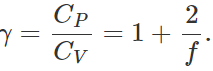Q.33 Can specific heat of a gas be negative?

Answer: Yes; negative specific heat will imply that with rise in temperature Undefined control sequence \Tau heat will be released(Q is - ve). This actually happens in case of saturated vapour i.e., specific heat of saturated vapour is negative.
Q.34 What is the specific heat of a gas in an isothermal process?
As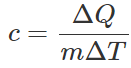For an isothermal process, ΔT=0, so c=∞.
Q.35 What is the specific heat of a gas in an adiabatic process?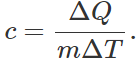For an adiabatic process, AQ = 0, so c= 0.
Q.36 A liquid is being converted into steam at its boiling point. What will be the specific heat of the liquid at this time?

Answer: During vaporisation, the temperature of the liquid remains constant (ΔT=0). Hence the specific heat, Undefined control sequence \Tau
Q.37 By what methods can the internal energy of an ideal gas be changed? Give examples.

Answer: The internal energy of an ideal gas can be changed by heating or cooling the gas in a closed vessel, by adiabatic compression or expansion of the gas.
Q.38 Heat equivalent to 50 joule is supplied to a thermodynamic system and 10 joule work is done on the system. What is the change in the internal energy of the system in the process?

∴   ΔU=ΔQ−ΔW=50−(−10)=+60J Thus internal energy of the system increases by 60J.
Q.39 400J of work is done on a gas to reduce its volume by compression adiabatically. What is the change in internal energy of the gas?

Answer: For an adiabatic process, ΔQ=0 As work is done on the gas, so  Δ W = - 400 J Change in internal energy, ΔU=ΔQ−ΔW=0−(−400) =+400J
Q.40 What is difference between heat and work?

Answer: Heat and work are two different modes of energy transfer to a system. Heat is the energy transfer that occurs due to temperature difference between the system and the surroundings. Work in the energy transfer that is brought about by other means, such as moving the piston of a cylinder containing the gas, by raising or lowering some weight connected to it.
Q.41 Why is the conversion of heat into work not possible without sink at lower temperature?

Answer: For converting heat energy into work continuously, a part of the heat energy absorbed from the source has to be rejected. The heat energy can be rejected only if there is a body, whose temperature is less than that of the source. This body at low temperature is called sink.
Q.42 Explain why it is impossible to design a heat engine with 100% efficiency. Or Even Carnot engine cannot have 100% efficiency. Explain, why?

Answer: Efficiency, η=1− T2/T1 The efficiency will be 100% or 1, if T2=0K. Since, temperature equal to 0 K cannot be realised, a heat engine with 100 efficiency cannot be designed.
Q.43 Why can a ship not use the internal energy of sea water to operate its engine?

Answer: For the operation of a heat engine we require a sink at temperature lower than the source and of sufficiently high thermal capacity, which is not possible in the sea.
Q.44 In a Carnot engine, the temperature of the sink is increased. What will happen to its efficiency?

Answer: Efficiency, η=1− T2/T1 If the temperature (T2) of the sink is increased, the efficiency of the Carnot engine will decrease.
Q.45 What is meant by reversible engine? Explain why the efficiency of a reversible engine is maximum?

Answer: The engine in which the process can be retraced at any stage of its operation by reversing the boundary conditions is called reversible engine. Its efficiency is maximum because in such a device no dissipation of energy takes place against friction, etc.
Q.46 The temperature of the surface of the sun is approximately 6000 K. If we take a big lens and focus the sun rays, can we produce a temperature of 8000 K?

Answer: No. According to second law of thermodynamics, heat by itself cannot flow from a body at lower temperature to a body at higher temperature. This can only be accomplished with the help of an external agency.
Q.47 Is the efficiency of a heat engine more in hilly areas than in the plains?

Answer: In hilly areas, the temperature of the surroundings is lower than that in plains, so the ratio T2/T1 is less in hilly areas than that in plains. But  η=1− T2/T1 Hence efficiency η is more in hilly areas than in plains.
Q.48 Is it theoretically possible to device a heat engine which will create no thermal pollution?

Answer: No. According to the second law of thermodynamics, whole of the heat cannot be converted into work. So a part of the heat that is not converted into work is exhausted by the engine to the atmosphere (sink). Hence thermal pollution will always occur.
Q.49 Can a kitchen be cooled by leaving the door of an electric refrigerator open? Or If a door of a working refrigerator is kept open for a long time in a closed room, will it make the room warm or cool?

Answer: No. A kitchen cannot be cooled by leaving the door of a refrigerator open, rather it will get slightly heated. When the door of the refrigerator is kept open, refrigerator now extracts heat from the kitchen room (acting as cooling chamber). Work is done on it by the electric motor and the total energy is rejected to the room (now acting as surroundings). Thus the work done by the motor gets added to the room, so it gets heated.
Q.50 How a refrigerator can be used as a heat pump to heat a house in winter?

Answer: When the outside environment is colder than the inside of a room, we leave a refrigerator open with its radiator (backside) facing the room. The refrigerator pumps in heat from the environment to the room. This heats up the room.
Q.51 Milk is poured into a cup of tea and is mixed with a spoon. Is this an example of a reversible process? Give reason.

Answer: No. When milk is poured into a cup of tea and mixed, some work is done which gets converted into heat. Heat produced cannot be converted back into work, which will separate milk from tea. Hence the mixing of milk with tea is an irreversible process.
Q.52 A thermos flask contains coffee. It is vigrously shaken. Consider the coffee as the system. (i) Has any heat been added to it? (ii) Has any work been done on it? (iii) Has its internal energy changed? (iv) Does its temperature rise?

Answer: (i) No. As the thermos flask is insulated, heat has not been added to the coffee (ΔQ=0).

(ii) Yes. Some work is done by the man in shaking the coffee against the forces of viscosity i.e., ΔW is negative.

(iii) By first law of thermodynamics, ΔQ=ΔU+ΔW. As ΔQ=0 and ΔW is negative, so ΔU is positive i.e., internal energy of the coffee increases.

(iv) Because of the increase in internal energy of the coffee, the temperature of the coffee will also increase.
Q.53 When a bore is made with a small drill in a hard board, the drill becomes very hot. But when the bore is made in a soft board, the drill does not become so hot. Why?

Answer: When a bore is made in a hard board, the friction between the drill and the board is quite large. A large amount of work has to be done in making the bore which produces a large amount of heat and the drill becomes too hot to touch. In case of the soft board, the friction is less, small work has to be done in making the bore. Hence heat produced is also small.
Q.54 What is an isothermal process? What are the essential conditions for an isothermal process to take place?

Answer: A process in which temperature remains constant is called isothermal process. The essential conditions for an isothermal process to take place are:

(i) The walls of the container must be perfectly conducting to allow free exchange of heat between the gas and the surroundings.

(ii) The process of compression or expansion should be slow, so as to provide sufficient time for the exchange of heat.
Q.55 What is an adiabatic process? What are the essential conditions for an adiabatic process to take place?

Answer: A process in which a thermally insulated system neither loses nor gains heat from the surroundings is called adiabatic process. The essential conditions for an adiabatic process to take place are:

(i) The walls of the container must be perfectly non conducting to prevent any exchange of heat between the gas and the surroundings.

(ii) The process of compression or expansion should be rapid, so that there is time for the exchange of heat.
Q.56 Explain why the internal energy of a compressed gas is less than that of a rarefied gas at the same temperature.

Answer: When a real gas is compressed, its molecules come closer. Their mutual interactions become stronger. This increases the magnitude of potential energy. But potential energy is negative. Hence the total energy of the compressed gas decreases. However, the kinetic energy is same for both the compressed and rarefied gases as both are at the same temperatures.
Q.57 When you whistle out air on to your palm held close to your mouth, the air feels cold; but when you blow out air from your mouth, keeping it wide open, the air feels hot. Why?

Answer: During whistling, we blow out air through a small opening between the lips. This is an adiabatic expansion, so there is a fall in temperature. But when we keep our mouth wide open, hot air of the mouth blows on to the palm which feels hot.
Q.58 Two bodies of specific heats c1 and c2 having same heat capacities are combined to form a single composite body. What is the specific heat of the composite body?

Answer: As the heat capacities are equal, so m1c1=m2c2. Let c be the specific heat of the composite body. Then (m1+m2)c=m1c1+m2c2=m1c1+m1c1=2m1c1 or

c=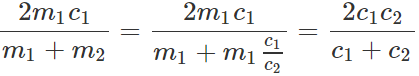Q.59 A gas expands in such a manner that its pressure and volume comply with the condition PV2=constant. Will the gas cool or get heated on expansion?

Answer: For a perfect gas, PV/T =constant (K) Given PV2=constant (K′) ∴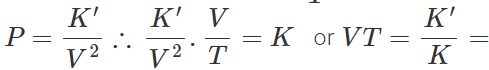constant Hence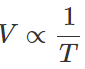That is the expansion of the gas will result in the decrease of temperature.
Q.60 What happens to the change in internal energy of a gas during (i) isothermal expansion and (ii) adiabatic expansion?

Answer: (i) Isothermal expansion. Temperature  Remains constant during an isothermal change. As internal energy is a function of temperature only, so it will remain constant during an isothermal change. As ΔT=0,so ΔU=CVΔT=0.

(ii) Adiabatic expansion. For an adiabatic change, ΔQ=0, so from first law of thermodynamics, ΔQ=ΔU+ΔW=0 or ΔW=−ΔU During expansion, work is done by a gas i.e., ΔW is positive. So ΔU must be negative. Hence internal energy of a gas decreases during an adiabatic expansion.
Q.61 No real engine can have an efficiency greater than that of a Carnot engine working between the same two temperatures. Give reason.

Answer: A Carnot engine is an ideal engine satisfying the following conditions:

(i) There is no friction between the walls of the cylinder and the piston.

(ii) The working substance is an ideal gas. That is the gas molecules have point sizes and have no attractive forces between them. Real engines cannot fulfill these conditions. Hence no heat engine working between the same two temperatures can have efficiency greater than that of a Carnot engine.
Q.62 Can a Carnot engine be realised in practice?

Answer: No. A Carnot engine should consist of

(i) a source of infinite thermal capacity,

(ii) a sink of infinite thermal capacity,

(iii) an ideal gas as its work substance. Moreover, the working substance should be contained in cylinder provided with frictionless, non-conducting movable piston. It is not possible to achieve all these conditions. Hence a Carnot engine cannot be realised in practice.
Q.63 Discuss whether the following phenomena are reversible : (i) Water fall (ii) Rusting of iron (iii) Electrolysis.

Answer: (i) Water fall. It is not a reversible process. During fall of the water, the major part of its potential energy is converted into kinetic energy of the water. However, on striking the ground, a part of it is converted into heat and sound. It is not possible to convert the heat and the sound produced along with the K.E. of water into potential energy so that the water may rise back to its initial height. Therefore, water fall is not a reversible process.

(ii) Rusting of iron. During rusting, iron gets oxidized by the oxygen of the air. Since it is a chemical change, it is not a reversible process.

(iii) Electrolysis. It is a reversible process, provided the electrolyte does not offer any resistance to the flow of current. If we reverse the direction of current, the direction of motion of ions is also reversed.

Offer running on EduRev: Apply code STAYHOME200 to get INR 200 off on our premium plan EduRev Infinity!

## Physics For JEE

188 videos|517 docs|265 tests

,

,

,

,

,

,

,

,

,

,

,

,

,

,

,

,

,

,

,

,

,

;